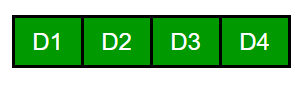# Count ‘d’ digit positive integers with 0 as a digit

• Difficulty Level : Easy
• Last Updated : 13 Jun, 2022

Given a number d, representing the number of digits of a positive integer. Find the total count of positive integer (consisting of d digits exactly) which have at-least one zero in them.
Examples:

```Input : d = 1
Output : 0
There's no natural number of 1 digit that
contains a zero.

Input : d = 2
Output : 9
The numbers are, 10, 20, 30, 40, 50, 60,
70, 80 and 90.```

## We strongly recommend that you click here and practice it, before moving on to the solution.

One Simple Solution is to traverse through all d digit positive numbers. For every number, traverse through its digits and if there is any 0 digit, increment count (similar to this).
Following are some observations:

1. There are exactly d digits.
2. The number at most significant place can’t be a zero (no leading zeroes allowed).
3. All the other places except the most significant one can contain zero .So considering the above points, let’s find the total count of numbers having d digits:

```We can place any of {1, 2, ... 9} in D1
Hence D1 can be filled in 9 ways.

Apart from D1 all the other places can be  10 ways.
(we can place 0 as well)
Hence the total numbers having d digits can be given as:
Total =  9*10d-1

Now, let's find the numbers having d digits, that
don't contain zero at any place.
In this case, all the places can be filled in 9 ways.
Hence count of such numbers is given by:
Non_Zero = 9d

Now the count of numbers having at least one zero
can be obtained by subtracting Non_Zero from Total.
Hence Answer would be given by:
9*(10d-1 - 9d-1) ```

Below is the program for the same.

## C++

 `//C++ program to find the count of positive integer of a` `// given number of digits that contain atleast one zero` `#include` `using` `namespace` `std;`   `// Returns count of 'd' digit integers have 0 as a digit` `int` `findCount(``int` `d)` `{` `    ``return` `9*(``pow``(10,d-1) - ``pow``(9,d-1));` `}`   `// Driver Code` `int` `main()` `{` `    ``int` `d = 1;` `    ``cout << findCount(d) << endl;`   `    ``d = 2;` `    ``cout << findCount(d) << endl;`   `    ``d = 4;` `    ``cout << findCount(d) << endl;` `    ``return` `0;` `}`

## Java

 `// Java program to find the count` `// of positive integer of a` `// given number of digits ` `// that contain atleast one zero` `import` `java.io.*;`   `class` `GFG {` `    `  `    ``// Returns count of 'd' digit ` `    ``// integers have 0 as a digit` `    ``static` `int` `findCount(``int` `d)` `    ``{` `        ``return` `9` `* ((``int``)(Math.pow(``10``, d - ``1``)) ` `                 ``- (``int``)(Math.pow(``9``, d - ``1``)));` `    ``}` `    `  `    ``// Driver Code` `    ``public` `static` `void` `main(String args[])` `    ``{` `        ``int` `d = ``1``;` `        ``System.out.println(findCount(d));` `        `  `        ``d = ``2``;` `        ``System.out.println(findCount(d));` `        `  `        ``d = ``4``;` `        ``System.out.println(findCount(d));` `        `  `    ``}` `}`   `// This code is contributed by Nikita Tiwari.`

## Python3

 `# Python 3 program to find the` `# count of positive integer of a` `# given number of digits that` `# contain atleast one zero` `import` `math`   `# Returns count of 'd' digit` `# integers have 0 as a digit` `def` `findCount(d) :` `    ``return` `9``*``((``int``)(math.``pow``(``10``,d``-``1``)) ``-` `(``int``)(math.``pow``(``9``,d``-``1``)));`     `# Driver Code` `d ``=` `1` `print``(findCount(d))` `    `  `d ``=` `2` `print``(findCount(d))`   `d ``=` `4` `print``(findCount(d))`     `# This code is contributed by Nikita Tiwari.`

## C#

 `// C# program to find the count` `// of positive integer of a` `// given number of digits ` `// that contain atleast one zero.` `using` `System;`   `class` `GFG {` `    `  `    ``// Returns count of 'd' digit ` `    ``// integers have 0 as a digit` `    ``static` `int` `findCount(``int` `d)` `    ``{` `        ``return` `9 * ((``int``)(Math.Pow(10, d - 1)) ` `                 ``- (``int``)(Math.Pow(9, d - 1)));` `    ``}` `    `  `    ``// Driver Code` `    ``public` `static` `void` `Main()` `    ``{` `        ``int` `d = 1;` `        ``Console.WriteLine(findCount(d));` `        `  `        ``d = 2;` `        ``Console.WriteLine(findCount(d));` `        `  `        ``d = 4;` `        ``Console.WriteLine(findCount(d));` `        `  `    ``}` `}`   `// This code is contributed by nitin mittal.`

## PHP

 ``

## Javascript

 ``

Output :

```0
9
2439```

Time Complexity : O(logn)

Auxiliary Space: O(1)